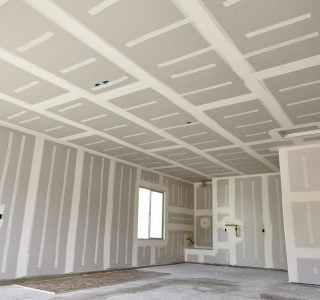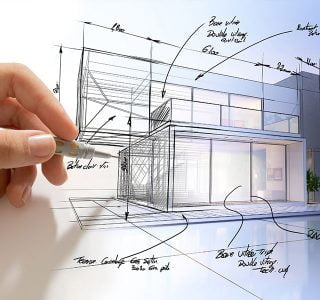Category: DrywallHow much does it cost to hang and finish drywall per square foot?

Drywall installation costs \$1.50 to \$3.50 per square foot, depending on the ceiling height, drywall type, and level of finish. Drywall costs \$0.50 to \$0.80 per square foot for materials. The labor cost to hang and finish drywall is \$1.00 to \$2.70 per square foot. Cost to Hang Drywall – Notes and General Information These estimates […]How do I calculate drywall installation?

Measure the total square footage of the areas where the drywall will be installed. Multiply the width times the height of each wall or ceiling area, then add all of the quantities together to get a total square-foot amount. How much does it cost per square foot to hang and finish drywall? Drywall installation costs \$1.50 to […]Everything You Should Know About Drywall Estimates

Calculate how many drywall sheets you will need by dividing the total square footage of area by 32 (if you’re using 4-by-8 sheets) or by 48 (if you’re using 4-by-12 sheets). If you want to get the cost, just multiply the total number of sheets by the price per sheet. Remember to add local taxes and delivery […]How do I calculate how much drywall I need?

Calculate how many drywall sheets you will need by dividing the total square footage of area by 32 (if you’re using 4-by-8 sheets) or by 48 (if you’re using 4-by-12 sheets). If you want to get the cost, just multiply the total number of sheets by the price per sheet. How much drywall do I need for […]How much drywall do I need for a 2,000 sq ft house

Our page on Average Drywall Installation Cost & Prices shows that the cost to drywall a house per square foot is roughly \$1.30 – \$4.00 per square foot. Average Cost to Drywall a House by House Size Cost to Drywall a 1000 sq ft House Total Cost Average x 6325 \$12,100 High x 7250 \$17,325 How […]

Get 20% off your first order

X